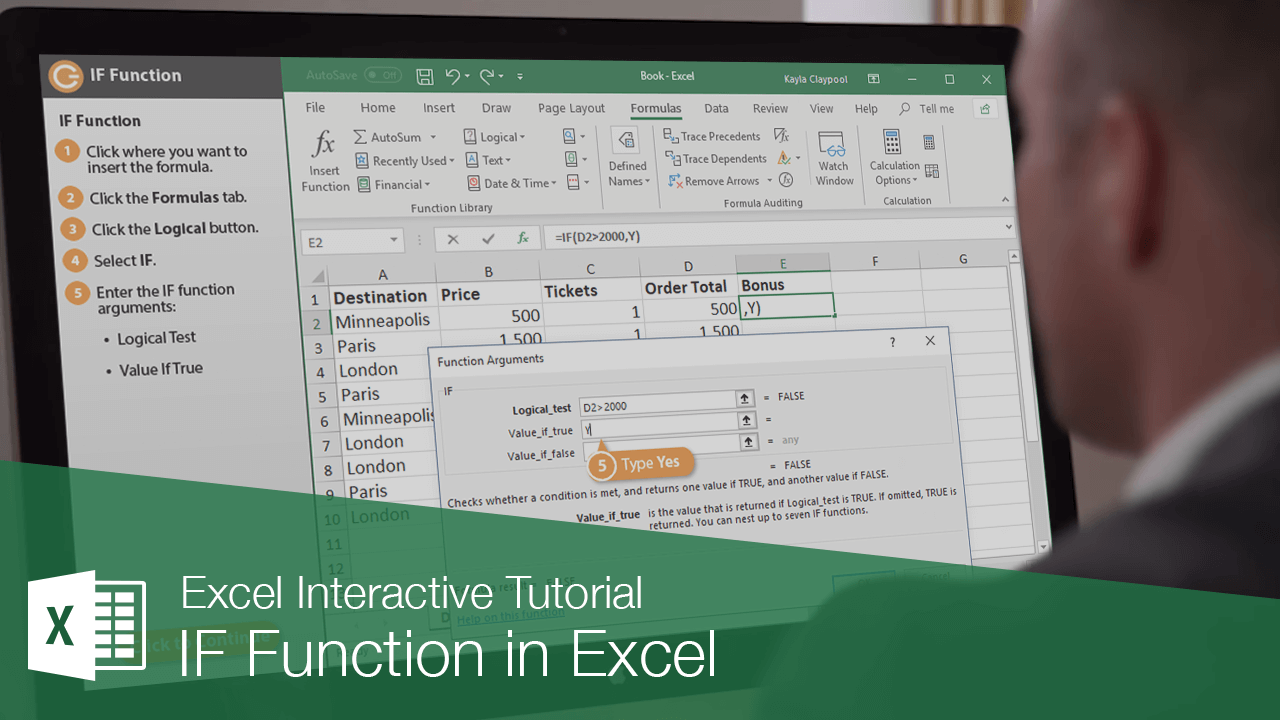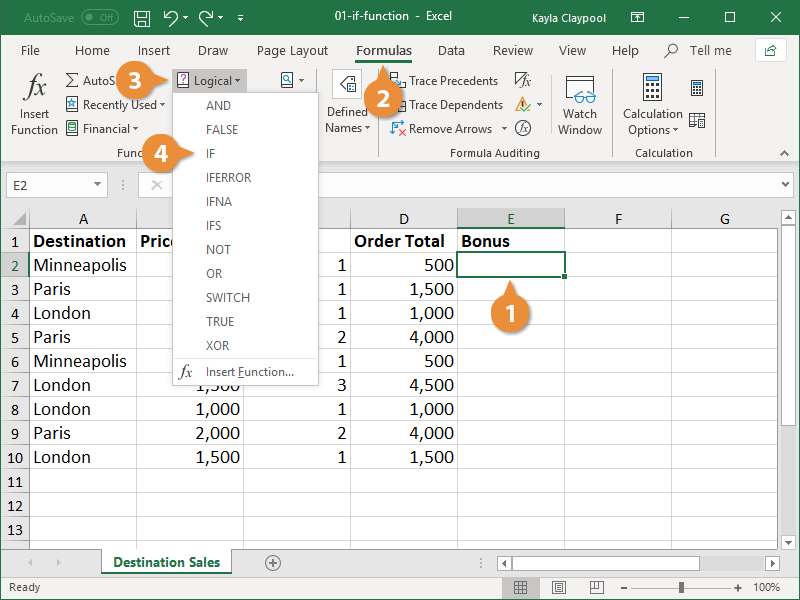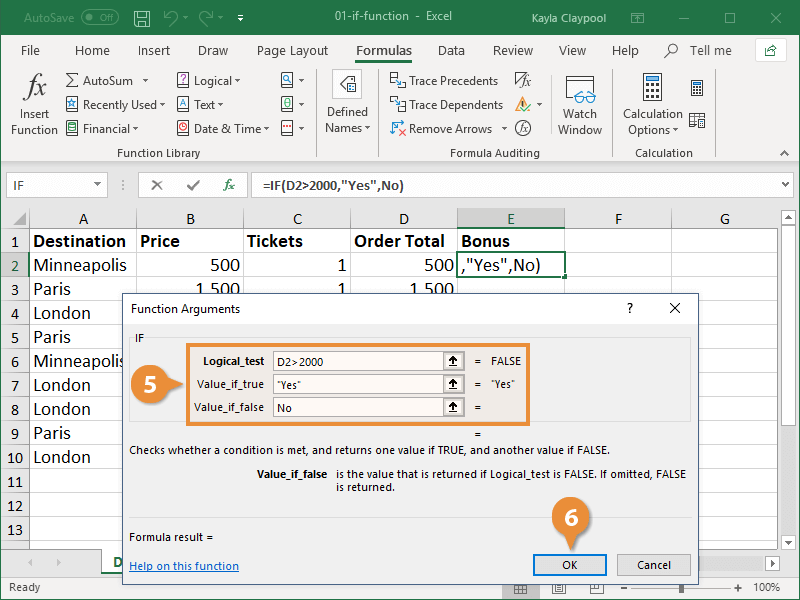How to use the IF Function in Microsoft Excel (Easy Guide) | CustomGuide# How to use the IF Function in Microsoft Excel (Easy Guide)

How to Use the IF Function in ExcelOne of the most popular functions in Excel is the IF function. The IF function evaluates a condition and returns one value if the condition is true and another value if the condition is false. For example, you could have Excel look at the values in a column of order totals and return Yes if a quota is met and No if a quota isn’t met.

The syntax for the IF function looks like this: =IF(logical_test, [value_if_true], [value_if_false]).

### Insert an IF Function

1. Click where you want to add the function.
2. Click the Formula tab.
3. Click the Logical button.
4. Select IF.The Function Arguments dialog box opens.

5. Enter the IF function arguments:
• Logical Test: Any value or expression that can be evaluated to TRUE or FALSE.
• Value If True: The value that is returned if Logical_test is TRUE. If omitted, TRUE is returned.
• Value If False: The value that is returned if Logical_test is FALSE. If omitted, FALSE is returned.
6. Click OK when you're finished.The IF function calculates and returns a value based on the evaluation of the logical test.

Note:You can nest up to seven IF functions in a given formula.